Next: 3.2.3 Simulations Up: 3.2 Extension of Neural Previous: 3.2.1 Algorithm

## 3.2.2 Alternative distance measure

For some applications, it proved to be of advantage to modify the error measure E(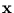). The error (3.2) depends on the size of the hyper-ellipsoid. Its volume is proportional to V =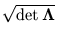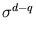. For a big ellipsoid, (3.2) has therefore a huge bias, namely 2 ln V. Thus, if the other ellipsoids are much smaller, this huge ellipsoid often has a high rank, independent of the Euclidean distance ofto the center of the ellipsoid. Since the weight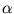decreases exponentially with the rank,is almost zero, and the ellipsoid cannot change its size anymore, it is dead' (for example, figure 3.8.A). Thus, to avoid dead units, we tested an alternative error measure that is independent of the volume of the ellipsoid. This can be achieved by normalizing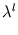and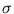such that the resulting volume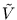is one. Let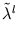and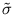be the normalized values. They can be computed by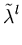=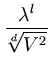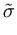=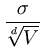. (3.9)

Using these substitutions, it can be straight-forwardly verified that the new volume=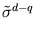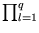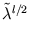fulfills= 1. To compute the modified error, we replaceandby their normalized values, and then we use the substitution (3.9) to obtain the error again in the original variables,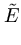(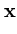) =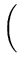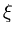T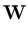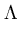-1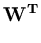+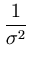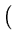T-T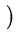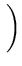V2/d . (3.10)

Here, the logarithm terms can be omitted because they are the same for all units. The modified algorithm uses (3.10) instead of (3.2) for the competition of the units. Everything else stays unchanged. The ellipsoids are still allowed to change their size according to the local PCA. In the following, this algorithm will be called NGPCA-constV', in contrast to NGPCA, which uses (3.2) for the competition.Next: 3.2.3 Simulations Up: 3.2 Extension of Neural Previous: 3.2.1 Algorithm
Heiko Hoffmann
2005-03-22